# AC Circuit

Direct current (DC) and alternating current (AC) are the two types of electricity. DC circuit and AC circuit show the structure of the respective circuit systems.

The main components of AC circuits are resistors, capacitors, and inductors.

All these passive electrical elements have one property in common; they restrict electric current in a circuit coil but in completely different ways.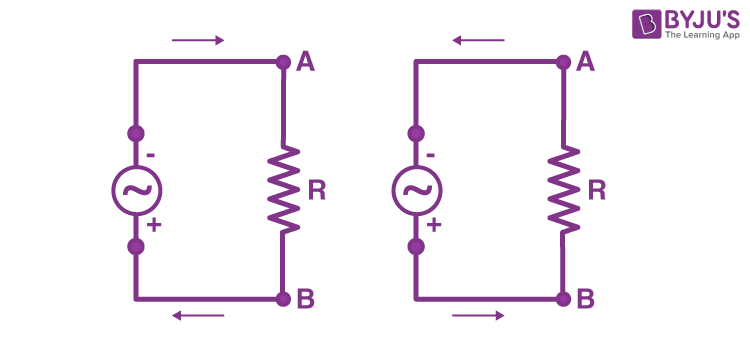The voltages and currents are marked by direction and magnitude in an AC circuit. The alternating values may or may not be in phase with each other, relying on the different variables of the system like capacitance, inductance and resistance. The sinusoidal alternating values are current and voltage, which differ by cohering to the sine of angle θ.

## AC Current

An alternating current (AC) is an electrical current that regularly reverses direction and changes its value constantly with time, contrary to DC current, which travels only in a single direction.

For many decades of electric power, the sinusoidal current and voltage have been used in power businesses and homes.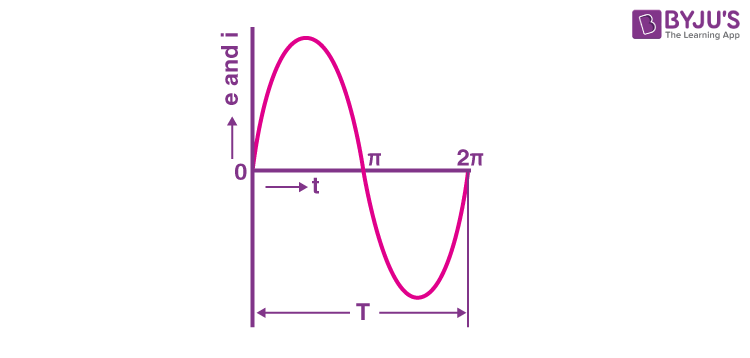### Various Terms Used in AC Circuits

• Amplitude
• Alternation
• Cycle
• Instantaneous Value
• Frequency
• Time Period
• Wave Form

## AC Circuit Types

There are different variations of AC circuits, such as AC circuits with only resistance (R), with only inductance (L), with only capacitance (C), the combination of RL, the combination of RC, the combination of LC and the combination of RLC.

### Resistor Capacitor (RC) AC Circuit

The RC circuit includes an AC generator, a resistor and a capacitor. A capacitor is fundamentally a group of conducting plates divided by an insulator. Thus, a stable current cannot flow through the capacitor. A time-changing current can subtract or add electrical charges from the capacitor plates.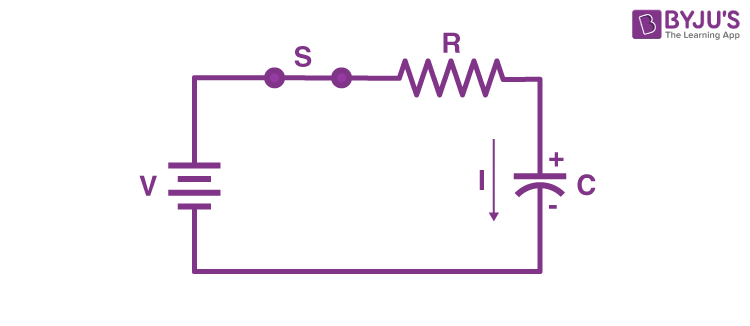### Resistor Inductor (RL) AC Circuit

The RL circuit includes an AC generator, a resistor and an inductor. When a circuit switch is activated in an RL AC circuit, a back EMF is generated in the inductor coil.

The current takes some time to achieve its peak value. The time constant is called the inductive time constant.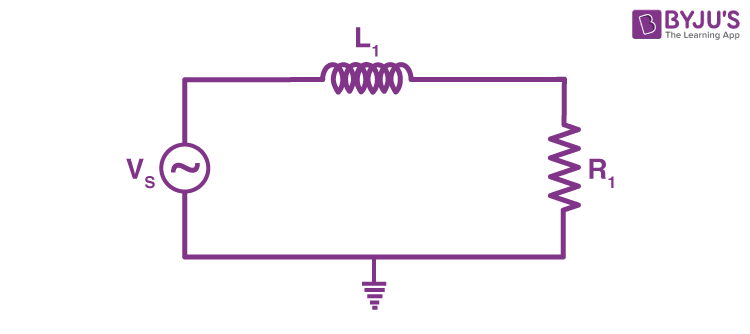A video about Charging by Induction.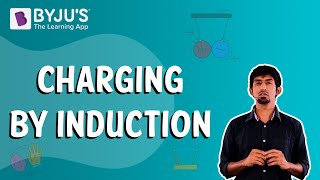### Resistive ( R ) AC Circuit

Resistors regulate, control, impede or set the current movement in a definite path or enforce a voltage cut in a circuit. Resistors have an impedance known as Resistance (R). The resistive value of a resistor is calculated in Ohms Ω. It can be calculated either in a locked value or a varying value (potentiometers).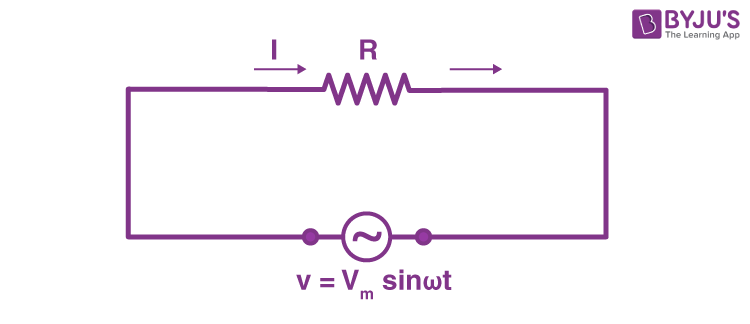### Resistor Inductor Capacitor ( RLC) AC Circuit

The RLC AC circuit is an AC circuit accompanying a resistor R, an inductor L, and a capacitor C, attached in a series or parallel.

The phase relation of these components can be stated as follows:

• The instantaneous voltage over the resistor is in phase with the instantaneous current.
• The instantaneous voltage over the inductor propels the instantaneous current by 90 degrees.
• The instantaneous voltage across the capacitor falls behind the instantaneous current.

Since the voltages over the various components are not in phase, each voltage cannot be simply summed up in AC circuits.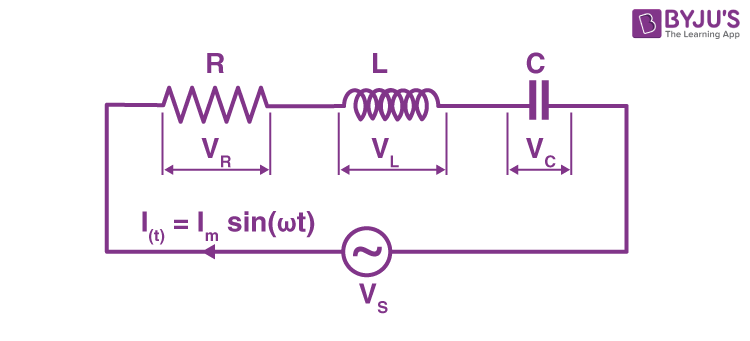Q1

### What are the main elements or components of an AC circuit?

The main components of AC circuits are resistors, capacitors, and inductors.

Q2

### How many switches are in a series DC circuit?

There is only one switch in a series AC circuit.

A single switch controls the entire series circuit. We cannot individually control each section of this type of circuit.

Q3

### What are the main types of AC circuits?

AC circuits can be divided into three categories: Resistive (R), RC, RL, and RLC circuits.

Q4

### What is a resistor?

It is a passive electrical unit with two terminals that are operated to either limit or regulate current movement in electrical circuits.

Q5

### What is a capacitor?

A capacitor is an electrical component that stores electric energy in an electrical field.

It is a passive electrical device with two terminals.

Q6

### What is an inductor?

An inductor is a two-terminal electronic element that accumulates energy in a magnetic field, when an electrical current passes through it.

Stay tuned to BYJU’S and Fall in Love with Learning!

Test your Knowledge on AC Circuit